## D-Flip Flops from Electronics Comp store don’t work

~ Sanjay Tumati

We have an electronics lab as part of STEM land and students who are interested including the old students who have studied in STEM land either at Isai ambalam school or Udavi come to the lab to learn and build things using electronics.  This blog is to share one of their experiments at the lab.

The students were excited as they were going to do an interesting experiment in the lab. One wave of the hand over a sensor would turn on an LED and another wave would turn it off. However, the entire experiment was ruined by faulty D Flip Flops (from now on referred to as DFFs). It was not a complete loss. The students learnt how to test a DFF (Reliability via connectivity and functionality via understanding a DFF

1. Connect the power supply and ground and observe the current display on the power supply. Some devices heated up immediately drawing hundreds of mA from the supply
2. Some devices started heating up on connecting Set and reset pins to ground
3. Some started heating up on connecting other connections
4. Students did connectivity checks between Set/reset/Clock/Data pins to supply and ground and found that in the faulty ones, the resistances were in the order of 100s of Ohms and not open circuit as was expected.
5. Just a couple of DFFs out of a dozen worked and those also went bad in a few minutes

This is the site from where we ordered the DFFs, Buy Electronics Components Online in India – Electronic Components Store

Surprising, because this site is usually very reliable and they send us quality components. We also ordered the CD4013 which is from the venerable CD40xx series. What did we do wrong with the DFFs? It can’t be that DFFs are uniquely susceptible to ESD handling. CD4017 and CD4033 which use several of these were just fine.

## How to Install WSL and Ubuntu (LTS) on the Windows platform

~Sandhiya.B and Ajay

This post consolidates the information needed to set up the Ubuntu terminal on a Win 11 Machine. The Ubuntu terminal is useful to programmers to access unix power tools, write shell scripts, etc.

Step: 1   Turn Windows Features On or Off

• Go to the search bar and type “Turn Windows features on or off” and open it.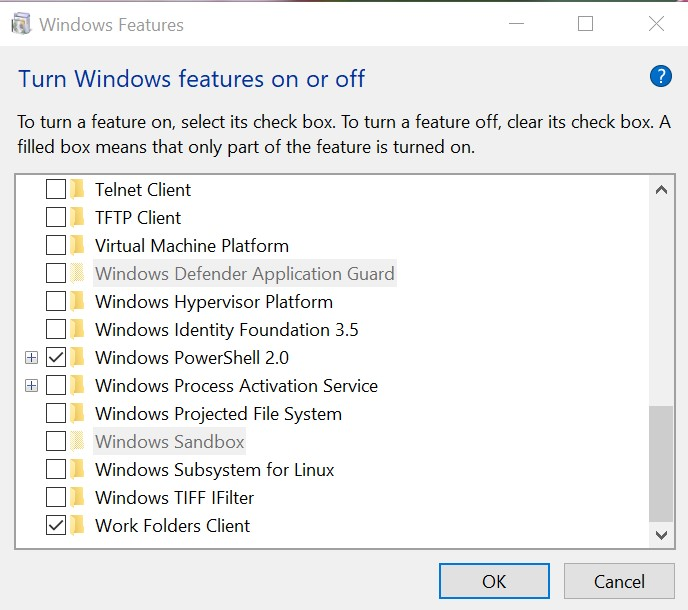• The check box is seen and find “Windows Subsystem for Linux” (if the check box is off, turn it on ) and also find “Virtual machine platform” (if the check box is off, turn it on ), And click the ok button.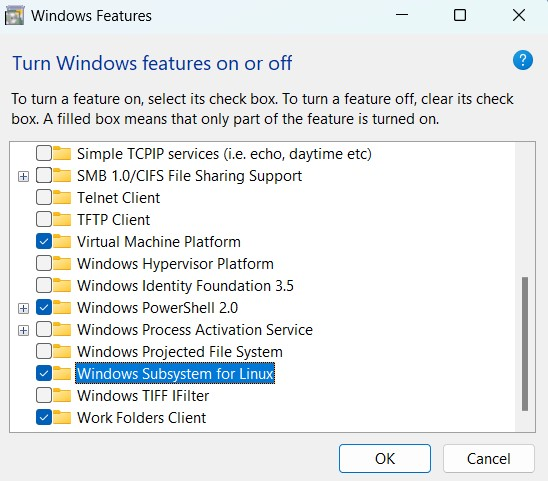• It requires restarting your system to click on the “Restart now” button.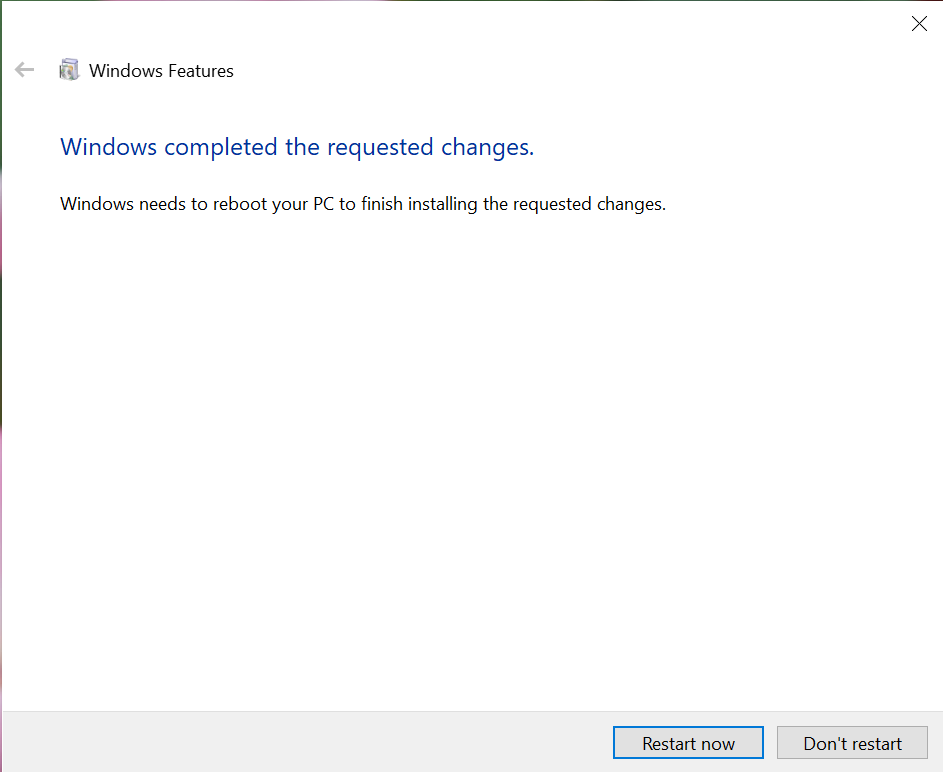Step: 2 Wsl Installations: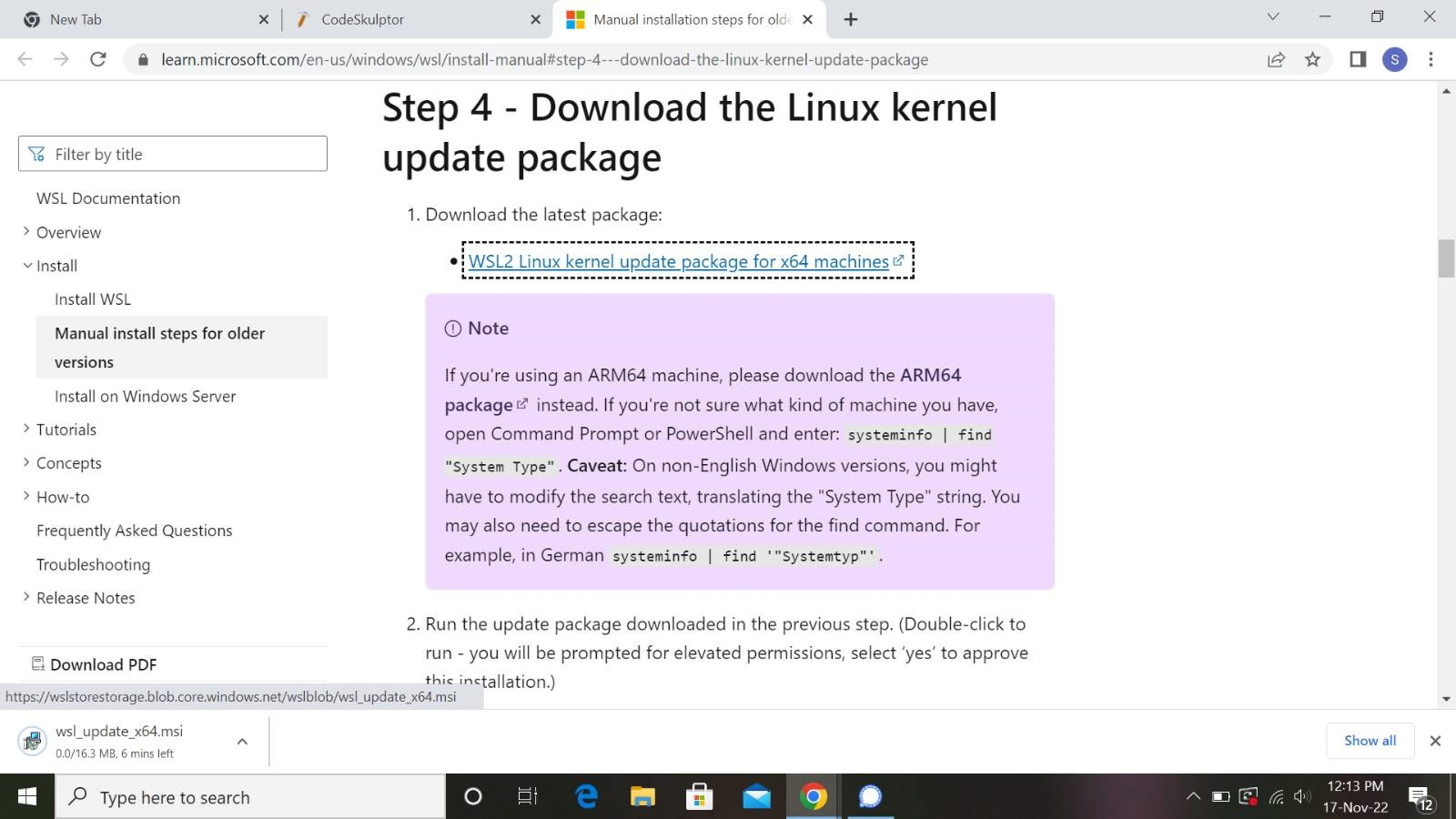Install Wsl [Windows Subsystem for Linux],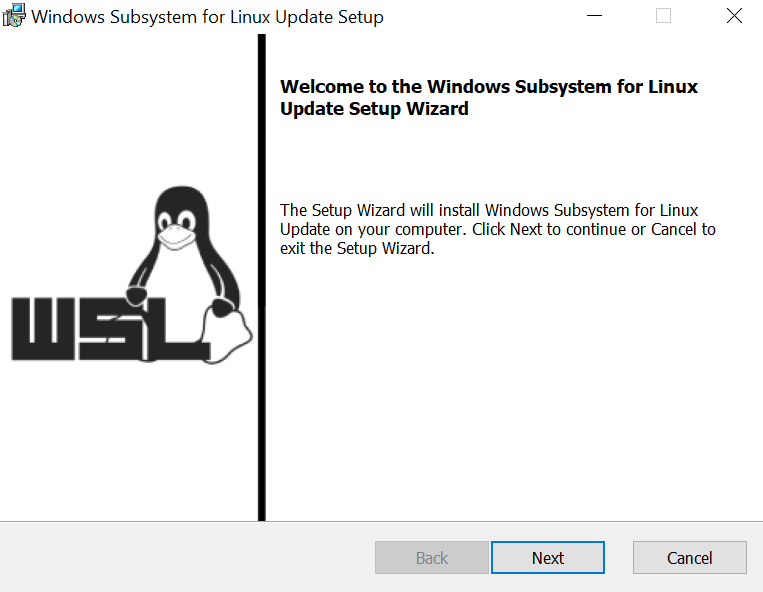• After installation click the “Finish” button.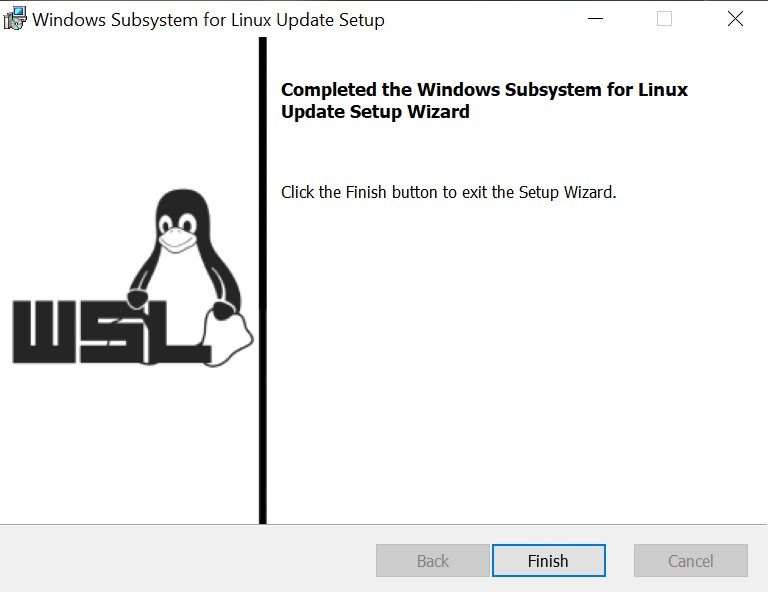Step: 3 Ubuntu Installations:

• Open the Microsoft Store (search for “store” from the start menu)
• Search the store for “Ubuntu” many versions are shown just install Ubuntu(recommended) or any versions that are needed for you.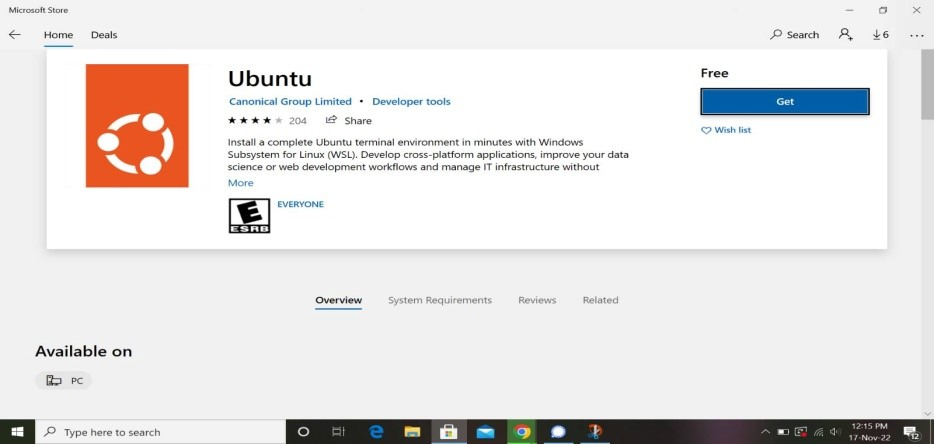• Click the “Get” or “Install” option.
• Once the download is completed click on the “Launch” or “Open” option.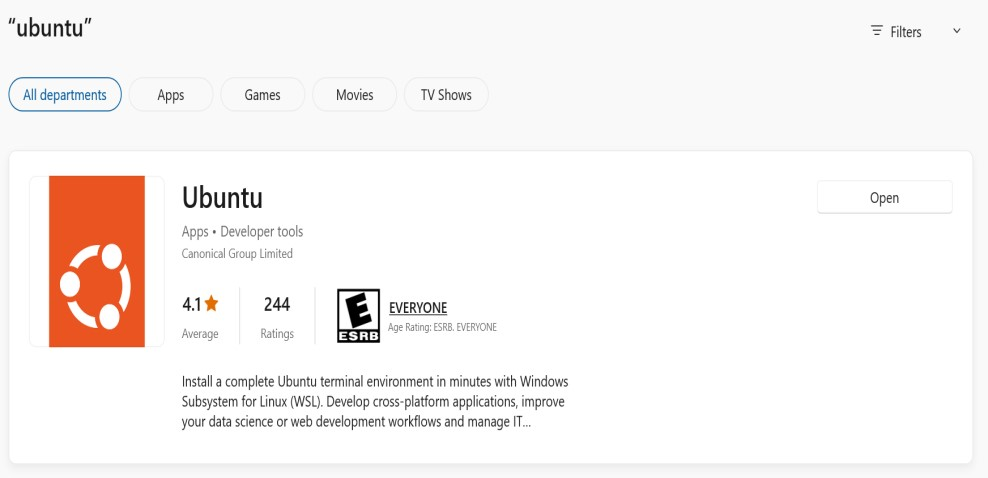• Once the ubuntu app is opened its interface is seen which is shown in the below image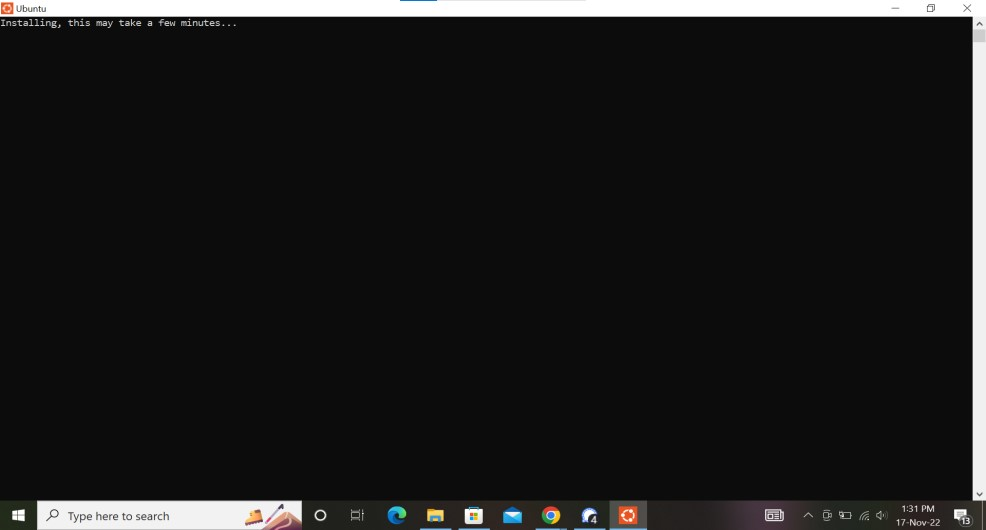• After installing enter the username and password for the ubuntu machine.
• NOTE:

(Username should be in lowercase and the Password need not be matched with windows)Step: 4 (if needed) to run on Windows terminal

• To run ubuntu on a windows terminal, First install the windows terminal in the Microsoft store,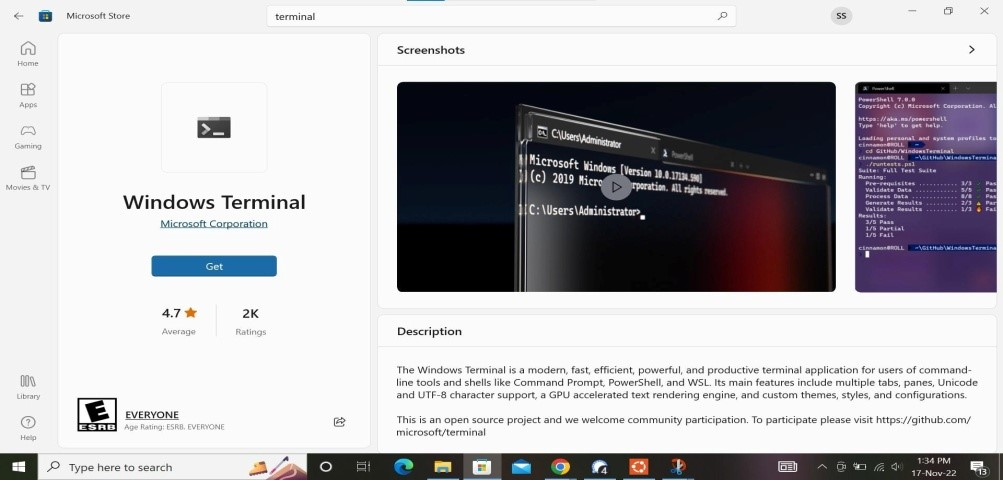• Click the “Get” or “Install” option
• Once the download is completed click on the “Launch” or “Open” option.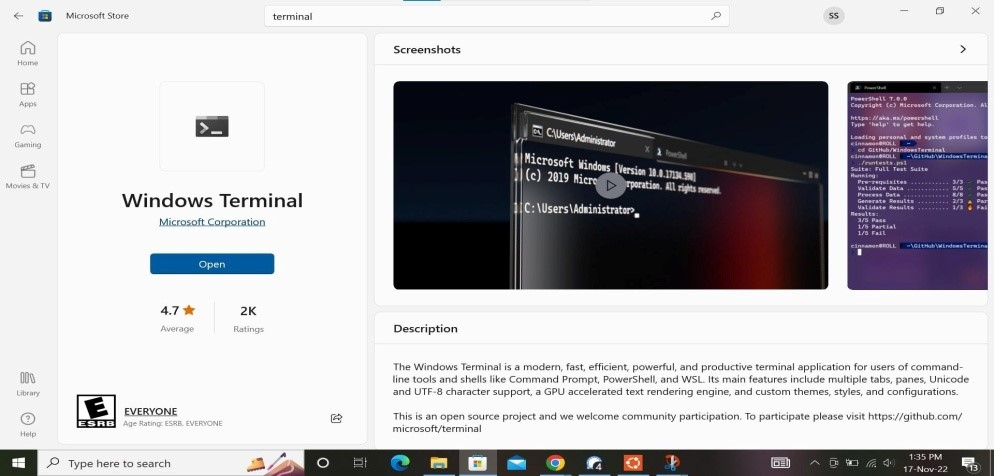• Once the Windows terminal app is opened its interface is like the below image,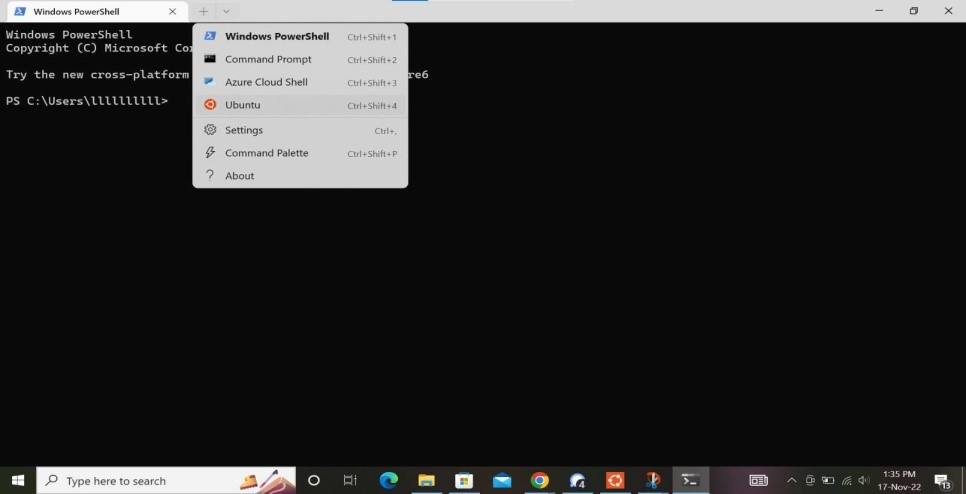• And choose the ubuntu terminal and its interface looks like the below image,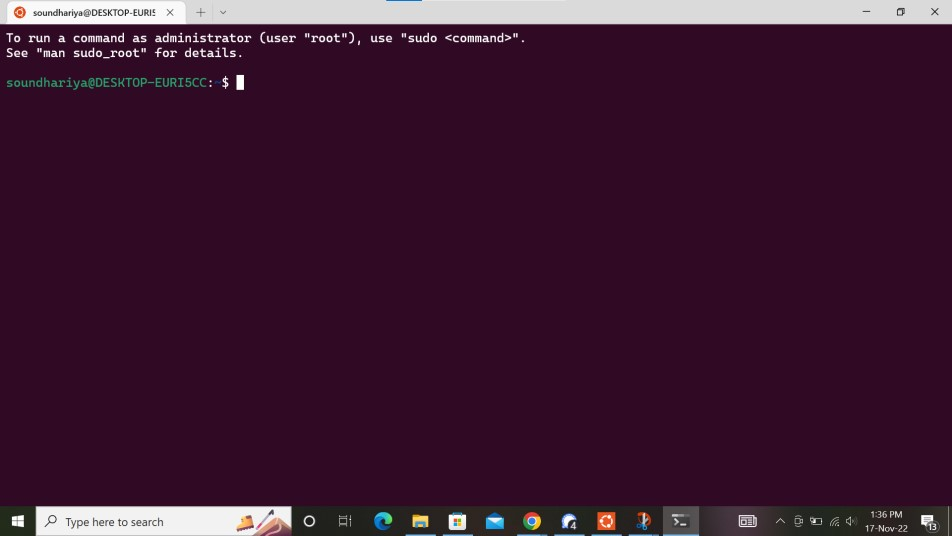• There you go! Now it’s ready for use.

In some exceptional cases there occurs some errors:

• The Error looks like this, the image given below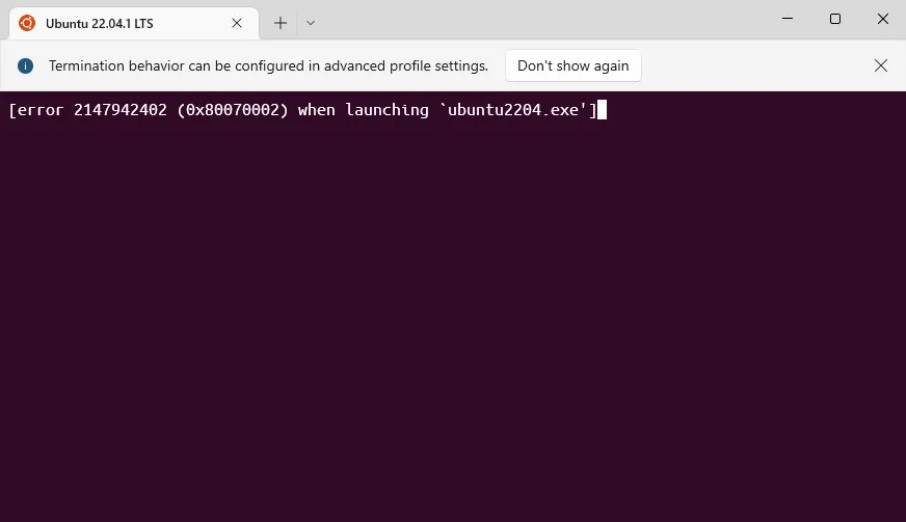• To solve this error, open file manager and go to local disk c.

Go to the user and user profile and go to App data (if not found, it is in hidden files) and go to the local folder and click on Microsoft file and go to windows apps and copy the path, which is shown below.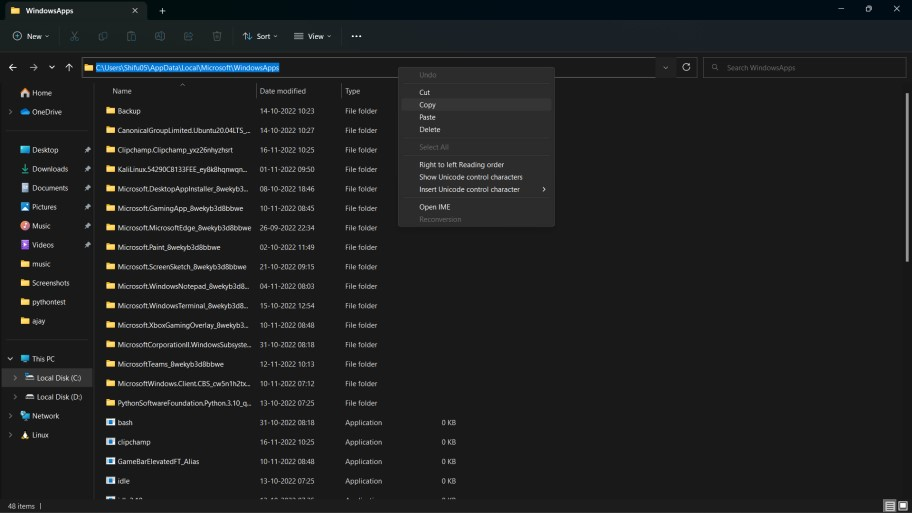• Go to the search bar and type “edit environment variables for your account” and open it, click on environment variable and double click on the path and Click on the new button paste the copied path and click ok,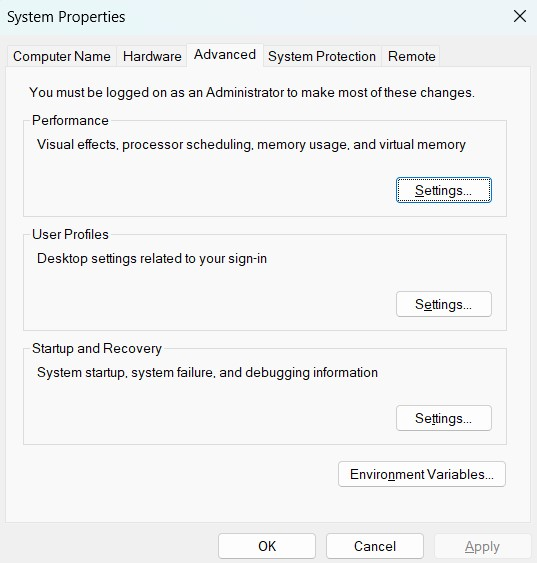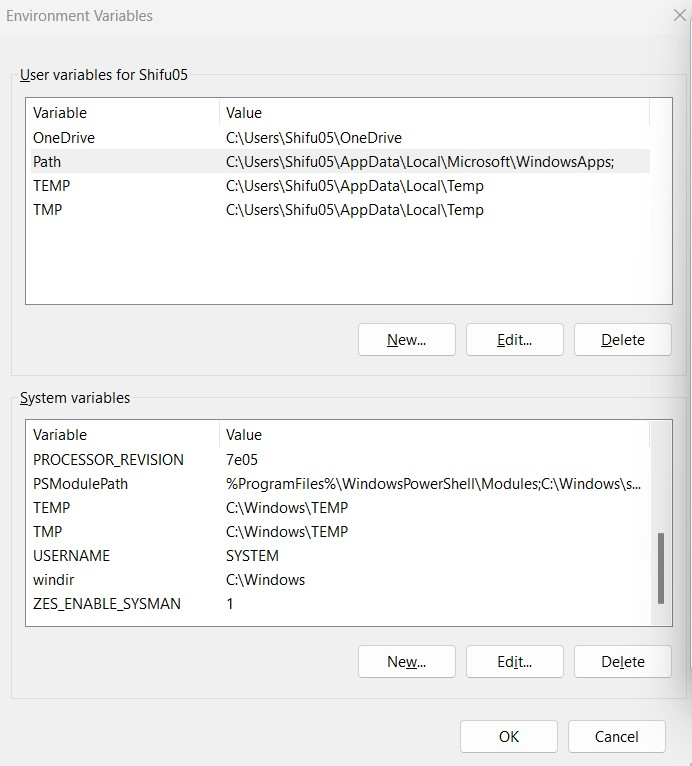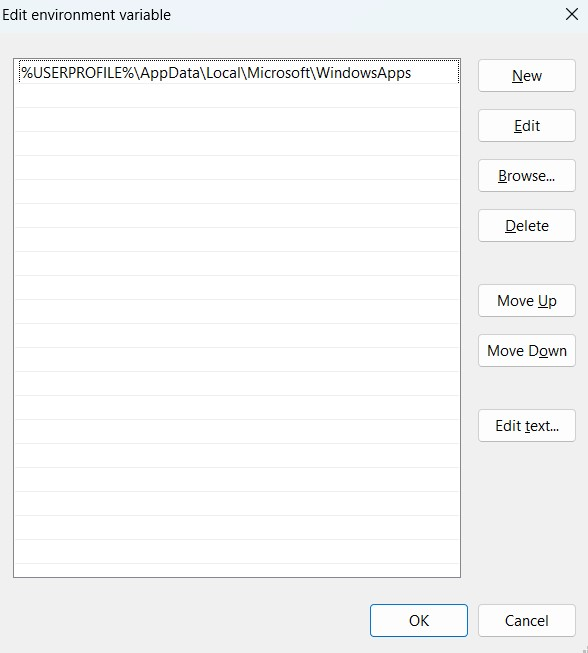• Now the error is fixed and the windows terminal can run the ubuntu machine.

## STEMland youths excel in CII PUDUCHERRY INNOVATION CONTEST 2022

The Confederation of Indian Industry (CII) works to create and sustain an environment conducive to the growth of the industry in India, partnering with industry and government alike through advisory and consultative processes. CII Puducherry Innovation Contest organized in partnership with Atal Incubation Centre, Pondicherry Engineering College Foundation is an ideal platform for the Students and Startupreneurs of Puducherry to showcase their innovative ideas, proof of concept, and prototypes. The event is structured through a 3-stage process that involves application shortlisting, online presentations, and a Pitch round. Boot camps, personal mentoring by Industrialists, and guidance by AIC are the salient features of the contest. Winners of the contest will get an opportunity to take their ideas to next level in the Atal Incubation Centre, PECF, and an opportunity to avail of seed funding.

The energetic youths of STEMland Vikinesh. R, Mugilan.M, and Punithavel.M from the Acharya College of Engineering and technology as the team participated in CII Puducherry Innovation Contest 2022 and presented their project “SALTWATER LAMP” and got selected for the finals of the Pitch round. They worked on this project to address the problems encountered by the fishermen and the public during disasters.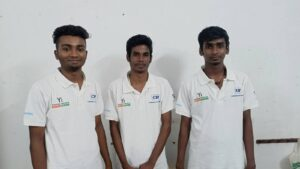From left, Vikinesh. R, Mugilan.M, and Punithavel.M.

First, they conducted a survey to formulate the objective and worked on a solution – saltwater lamps. They used the STEMland tinkering lab to develop this project. This project paved the way to help fishermen during the night-time, deep sea fishing, and the public during disasters. The project employed salt water to produce electricity through which the lamp glows. The team presented the project to the C3streamland team to have a final rehearsal before presenting in the contest and took feedback for growth.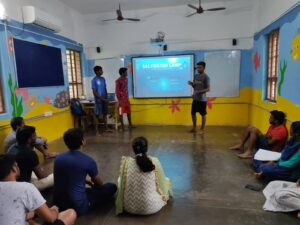The project set out to be a benchmark and inspired many rural youths to use the STEMland tinkering lab for developing projects.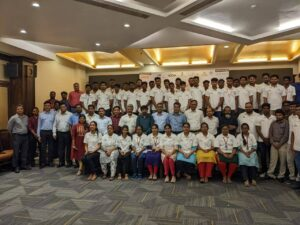## Visualizing trigonometric ratios using scratch program

~ Soundhariya, Sandhiya.B

Trigonometric ratios are the ratios of the length of sides of a triangle. These ratios in trigonometry relate the ratio of sides of a right triangle to the respective angle. The basic trigonometric ratios are sin, cos, and tan, namely sine, cosine, and tangent ratios. The other important trig ratios, cosec, sec, and cot, can be derived using the sin, cos, and tan respectively. Using scratch, the visualization of trigonometric ratios is comprehendible.

Let us have a look at the right-angled triangle drawn using the scratch shown below. Trigonometric ratios can be used to determine the ratios of any two sides out of a total of three sides of a right-angled triangle in terms of the respective angles.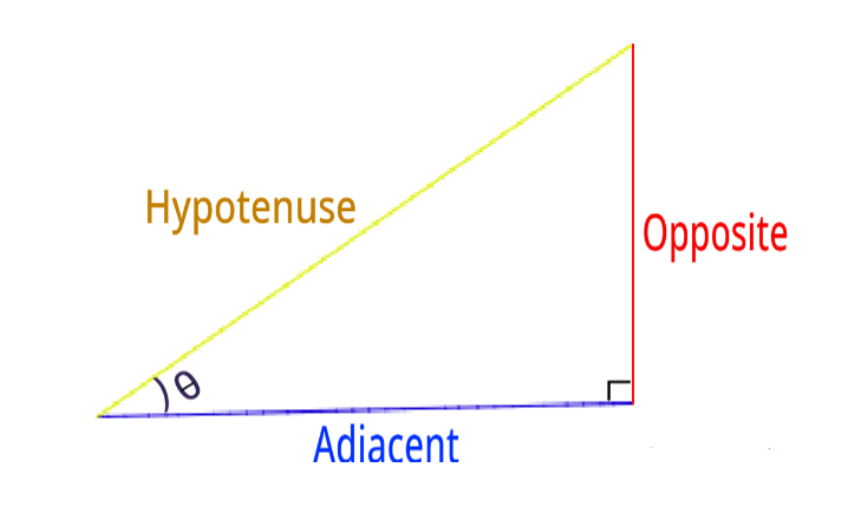The values of these trigonometric ratios can be calculated using the measure of an acute angle, θ in the right-angled triangle given below. This implies that the value of the ratio of any two sides of the triangle here depends on the angle. We can alternatively find the values of these trig ratios. Also, only the base and perpendicular will interchange for the given right triangle in that case.

Concerning θ, the ratios of trigonometry are given:

Sine: Sine of an angle is defined as the ratio of the side opposite (perpendicular side) to that angle to the hypotenuse.

cosine: The cosine angle is defined as the ratio of the side adjacent to that angle to the hypotenuse.

Tangent: The tangent angle is defined as the ratio of the side opposite to that angle to the side adjacent to that angle.

Cosecant: Cosecant is a multiplicative inverse of sine.

Secant: Secant is a multiplicative inverse of cosine.

Cotangent: Cotangent is the multiplicative inverse of the tangent.

The above ratios are abbreviated as sin, cos, tan, cosec, sec, and tan respectively in the order they are described. So, for Δ ABC, the ratios are defined as:

sin θ = (Side opposite to θ)/(Hypotenuse) = AB/AC

cos θ = (Side adjacent to θ)/(Hypotenuse) = BC/AC

tan θ = (Side opposite to θ)/(Side adjacent to θ) = AB/BC = sin ∠C/cos ∠C

cosec θ = 1/sin θ = (Hypotenuse)/ (Side Opposite to θ) = AC/AB

sec θ = 1/cos θ = (Hypotenuse)/ (Side Opposite to θ) = AC/BC

cot θ = 1/tan θ = (Side adjacent to θ)/(Side opposite to θ)= BC/AB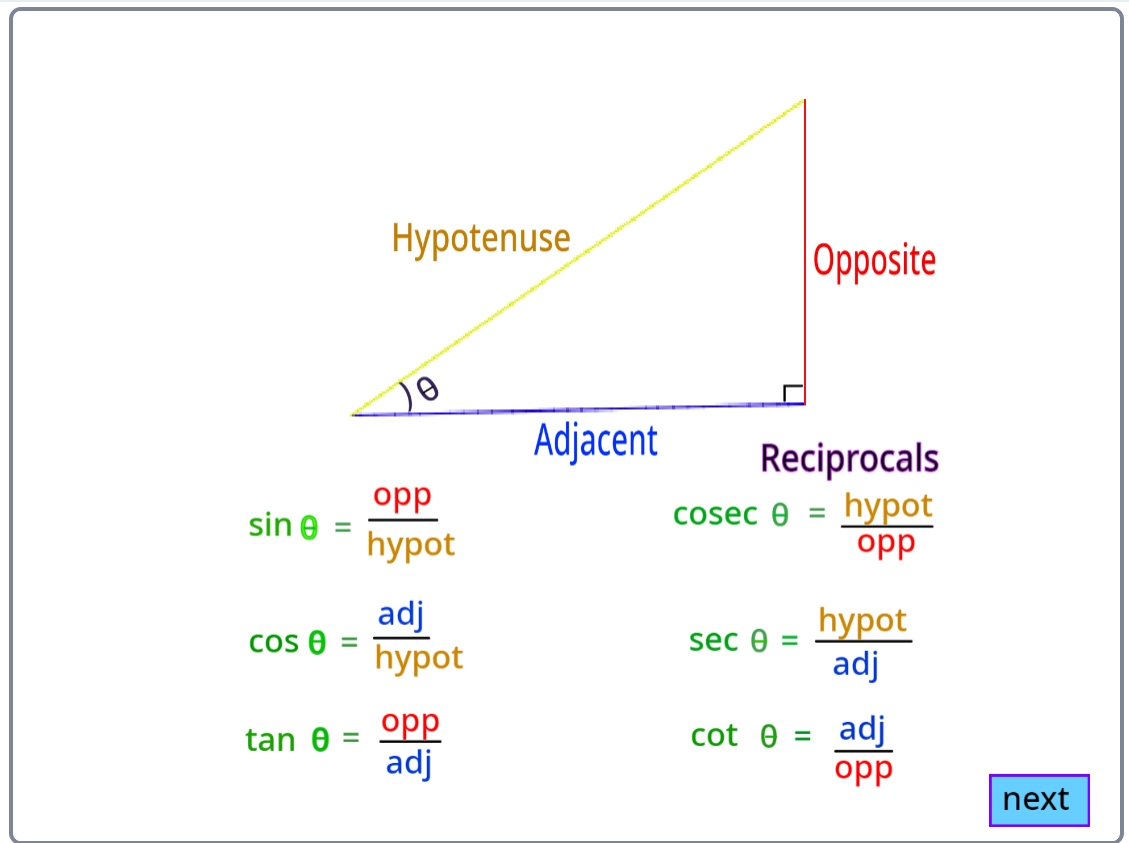Unit Circle and Trigonometric Values:

Unit circles can be used to calculate the values of basic trigonometric functions- sine, cosine, and tangent. The following diagram shows how trigonometric ratios can be represented in a unit circle.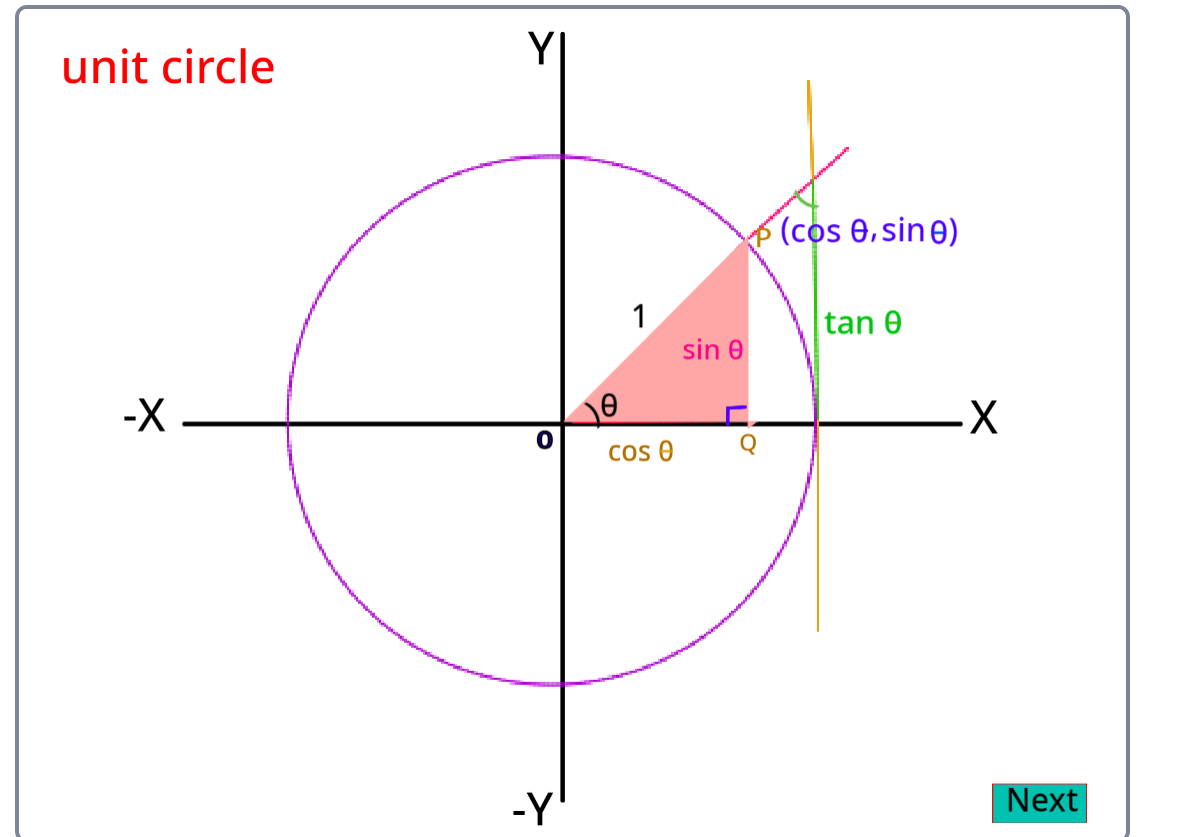In geometry, both degree and radian represent the measure of an angle. One complete anticlockwise revolution can be represented by 2π (in radians) or 360° (in degrees). Therefore, degree and radian can be equated as:

2π = 360° And π = 180°

Hence, from the above equation, we can say, 180 degrees is equal to π radian.

Usually, in general geometry, we consider the measure of the angle in degrees (°). Radian is commonly considered while measuring the angles of trigonometric functions or periodic functions. Radians are always represented in terms of pi, where the value of pi is equal to 22/7 or 3.14.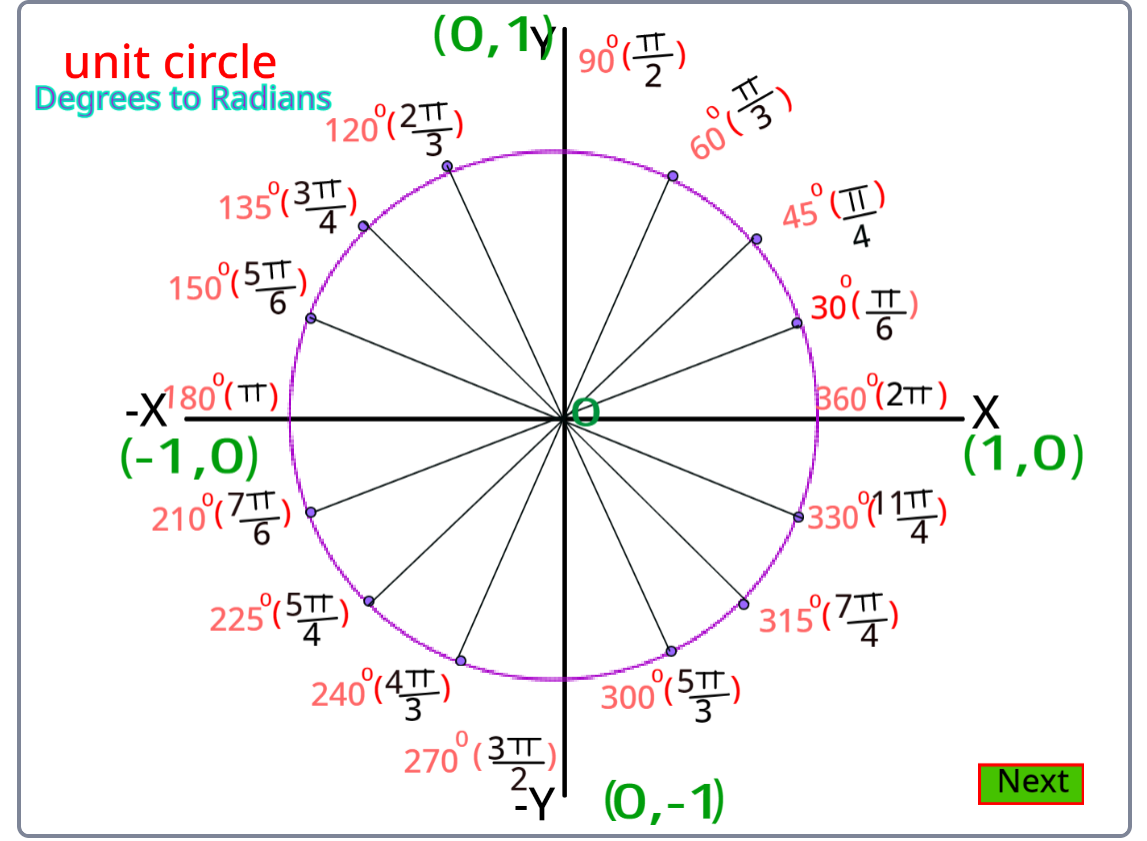Trigonometric ratios of some special angles:

In the trigonometric ratios table, we use the values of trigonometric ratios for standard angles 0°, 30°, 45°, 60°, and 90º. It is easy to predict the values of the table and to use the table as a reference to calculate values of trigonometric ratios for various other angles, using the trigonometric ratio formulas for existing patterns within trigonometric ratios and even between angles. The trigonometric ratios of 45° using scratch are shown below.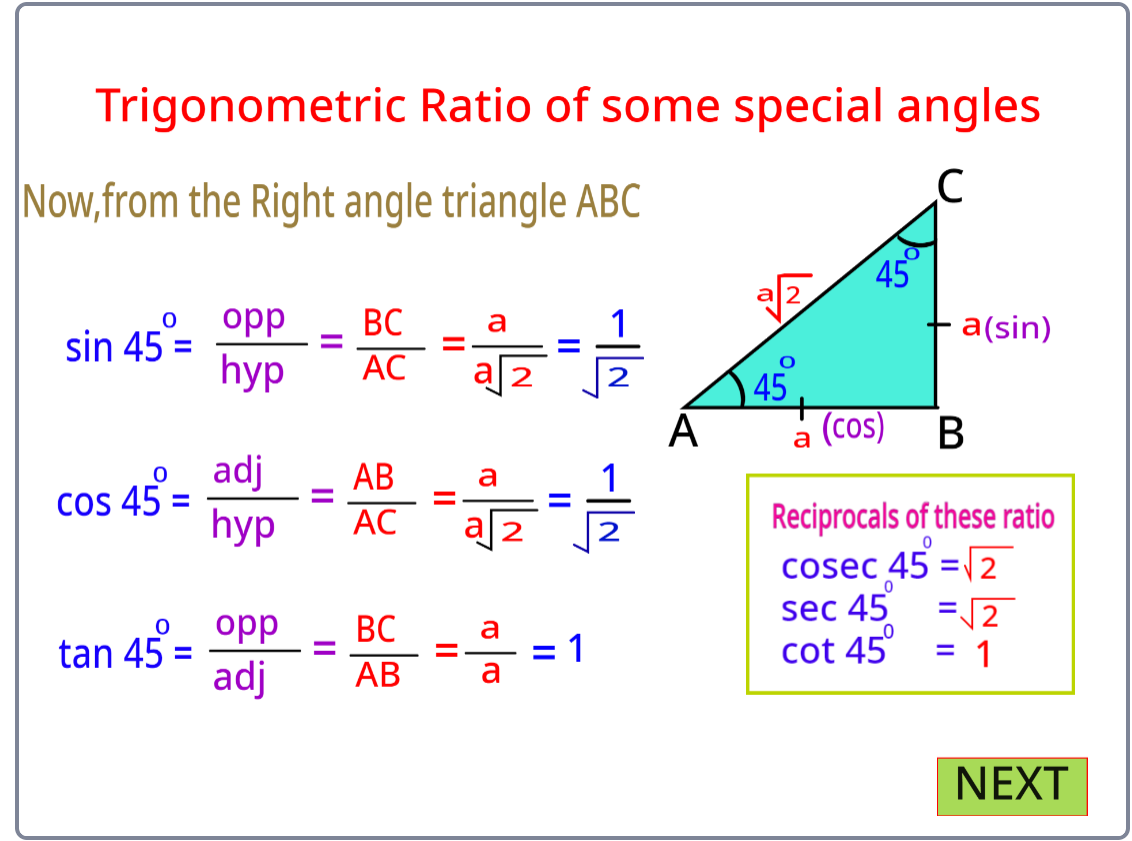In right Δ PQR, if ∠P and ∠Q are assumed as 30° and 60°, then there can be infinite right triangles with those specifications but all the ratios written above for ∠P in all of those triangles will be the same. So, all the ratios for any of the acute angles (either ∠P or ∠Q) will be the same for every right triangle. This means that the ratios are independent of the lengths of the sides of the triangle.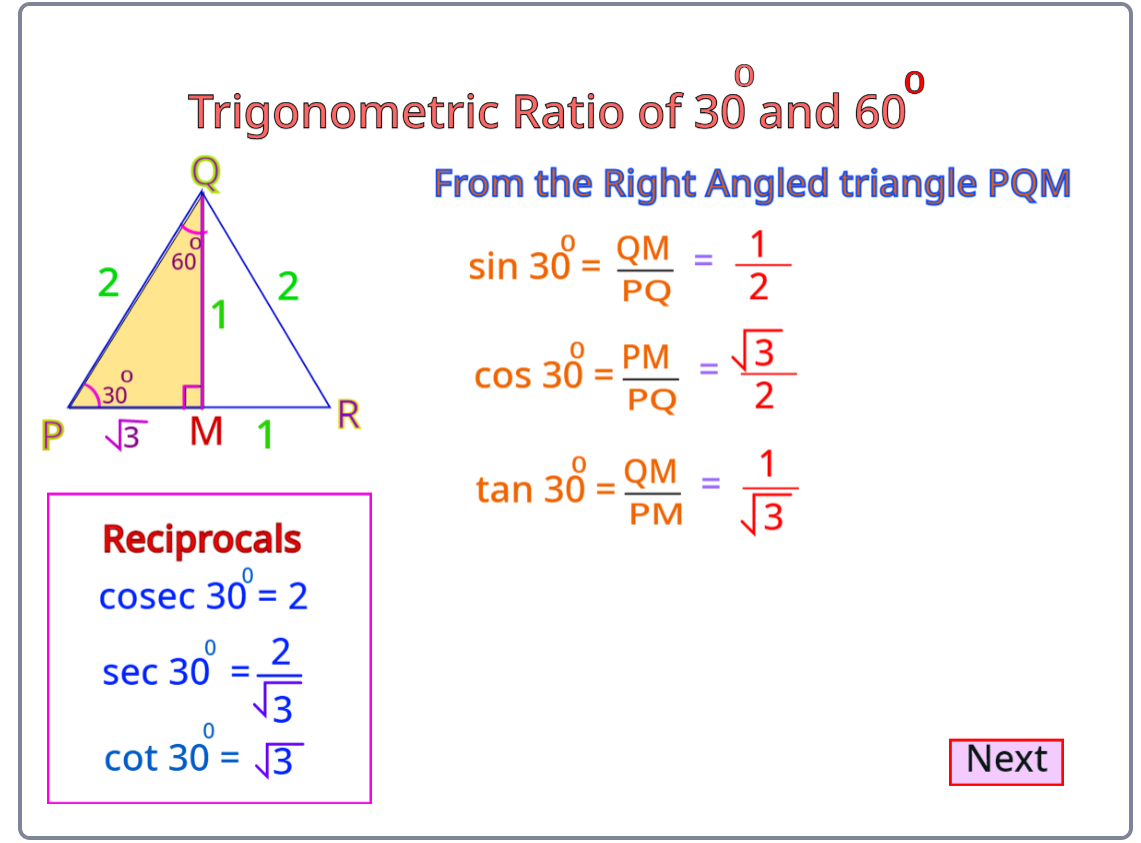The trigonometric ratios of 30° and 60°simulated using the scratch program are shown below.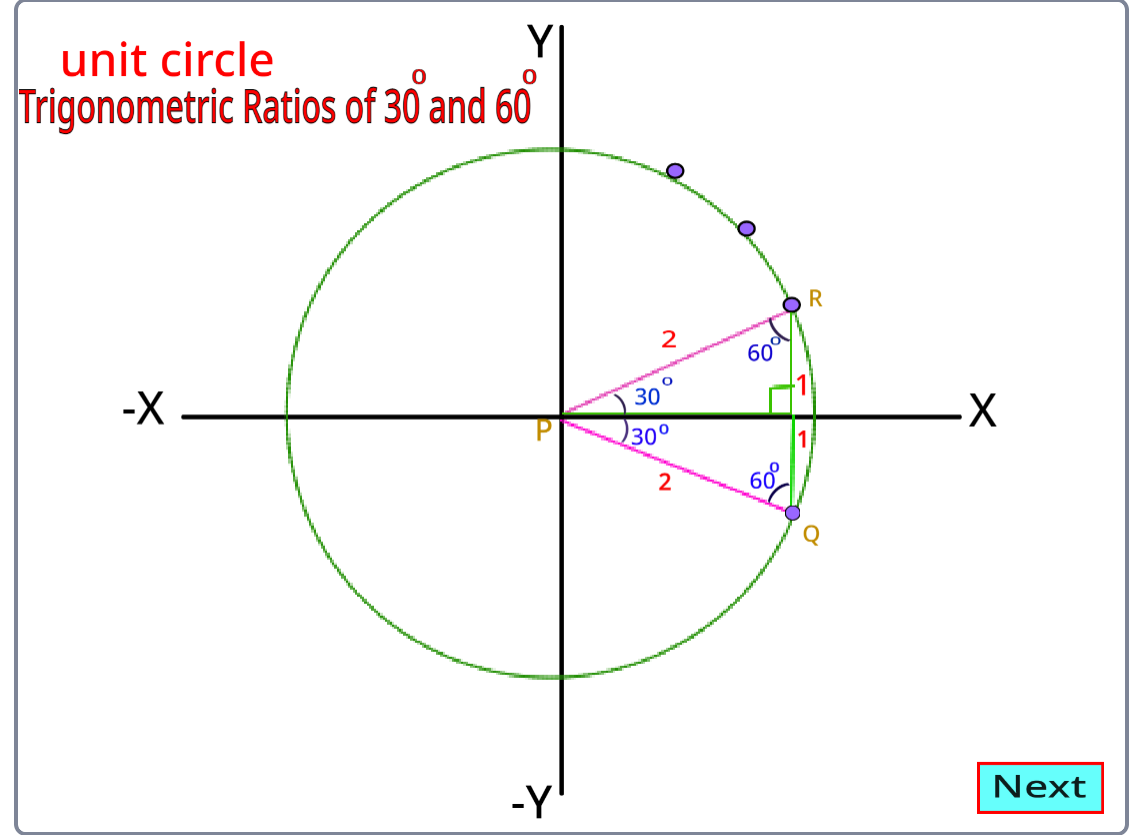The trigonometric ratios of 90° and 0° using scratch programming are shown below.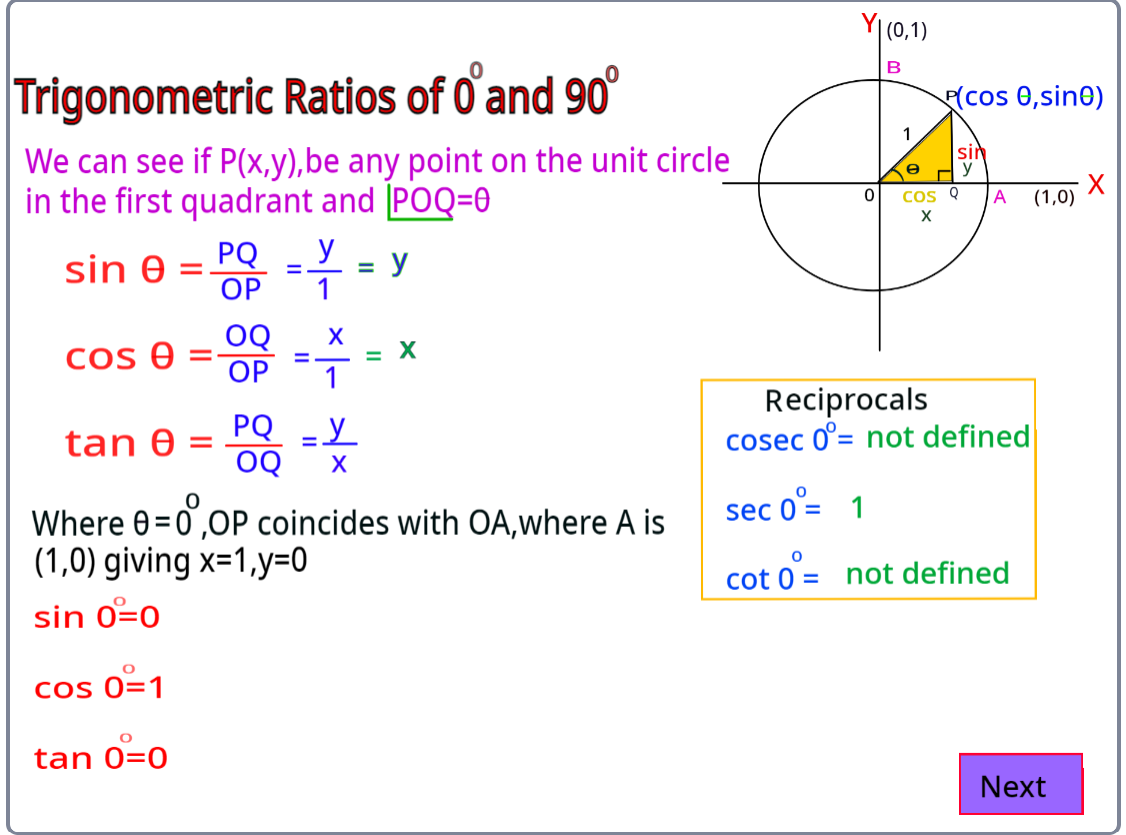Trigonometrical Ratios of 0 degrees are commonly called standard angles and the trigonometrical ratios of these angles are frequently used to solve particular angles. In ∆ABC is a right-angled triangle. If the length of the side BC is continuously decreased, then the value of ∠A will keep on decreasing. Similarly, the value of ∠C is increasing as the length of BC is decreasing. When BC = 0, ∠A = 0 , ∠C = 90° and AB = AC.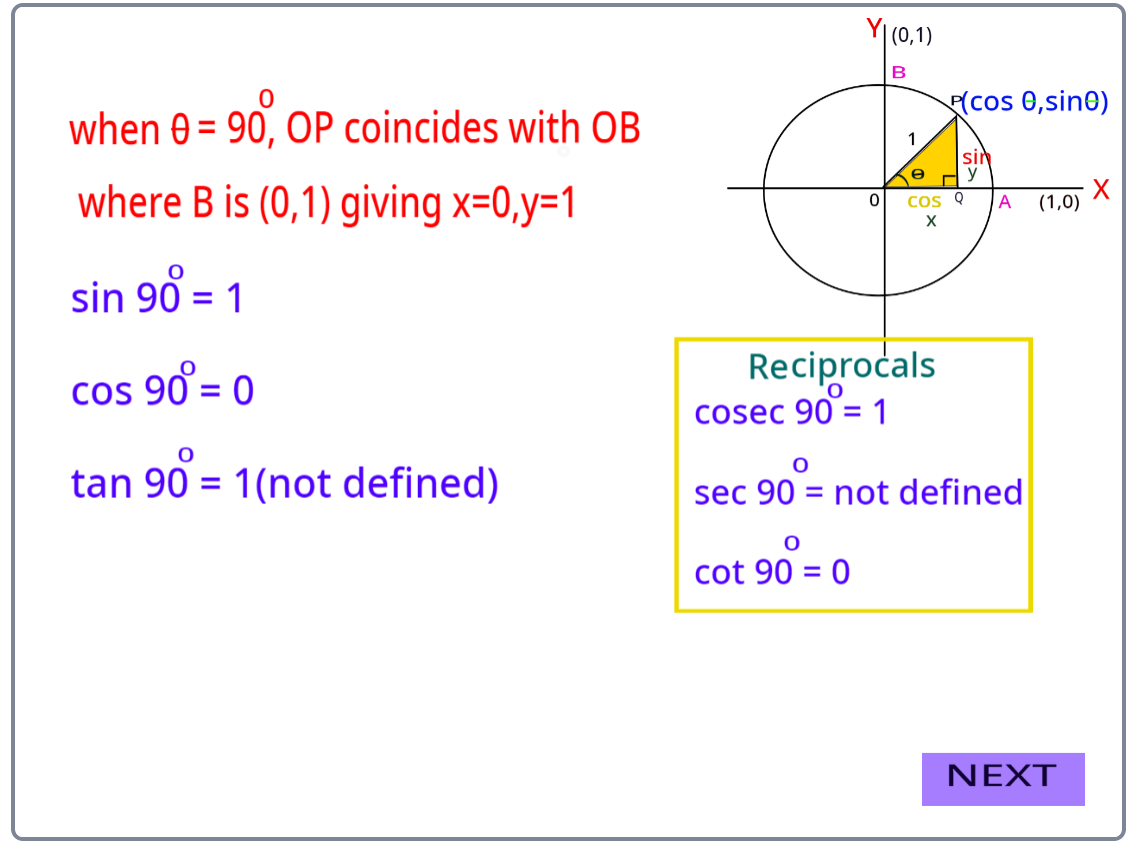Trigonometric Ratios Table:

The summarization of the value of trigonometric ratios for specific angles is in the table below: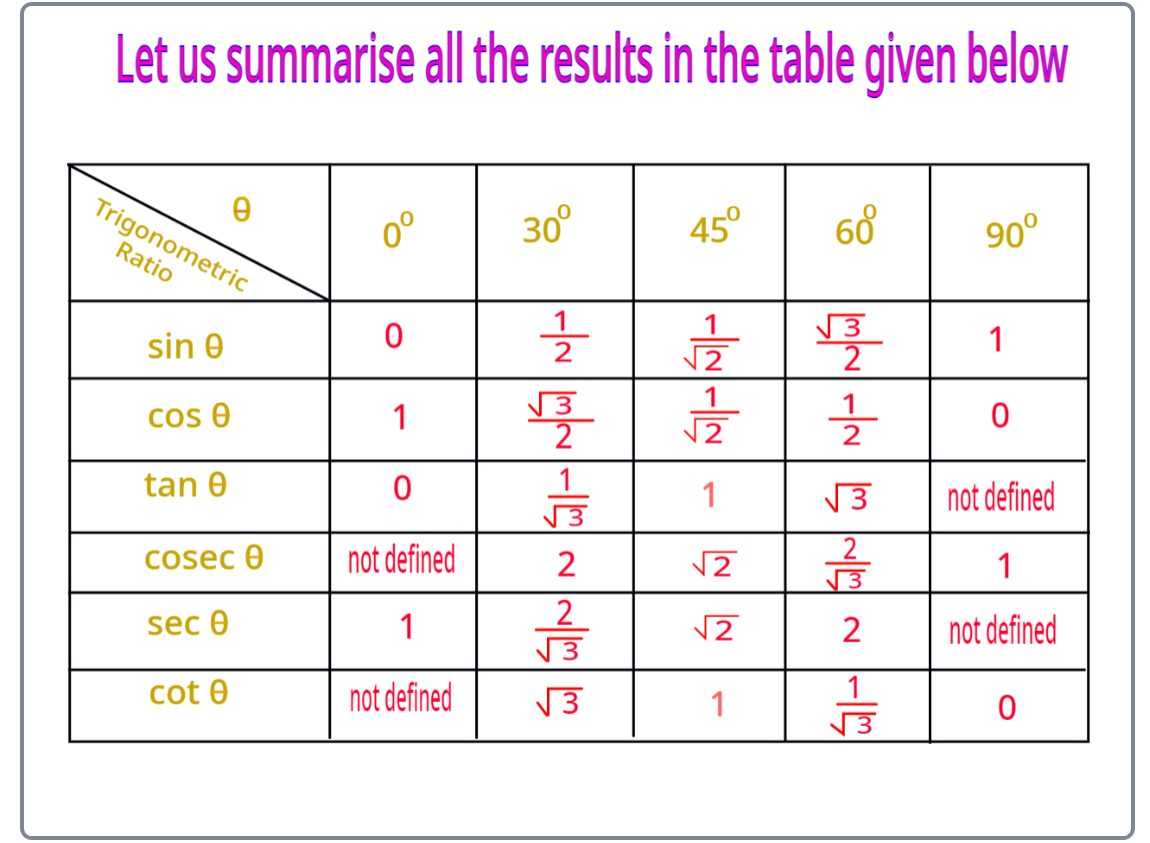Some of the applications of trigonometric ratios are:

• Measuring the heights of towers or big mountains
• Determining the distance of the shore from the sea
• Finding the distance between two celestial bodies
• Determining the power output of solar cell panels at different inclinations
• Representing different physical quantities such as mechanical waves, electromagnetic waves, etc.